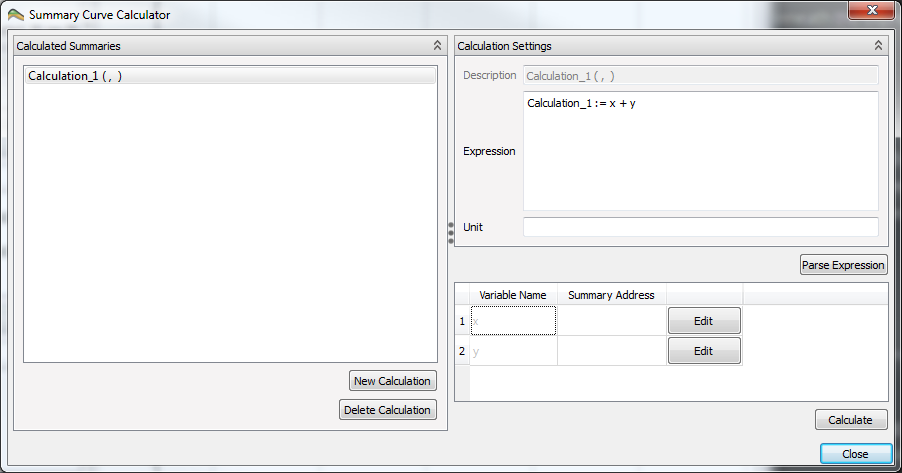# Curve CalculatorThe summary curve calculator is a tool to do relative simple vector calculations on a set of curves. The created curves can be stored for later use in the project.

The calculator can be run by pressing the calculator iconin the menu bar, or by right-clicking on either a summary case or the summary plot collection.

## Calculation Settings

To make a new calculated curve, click on New Calculation. This will add a new calculation to Calculated Summaries. Before choosing which curves to do calculations on, a calculation expression must be made. The default expression Calculation_1 := x + y will do a vector addition on the curves which x and y are placeholders for, and assign it to the calculation Calculation_1. How to assign curves to assign to x and y will be covered in section Summary Address Selection.

### Operators and Functions

Possible operations and functions are found by right-clicking in the expression window. The following tables show all the options available.

#### Assignment Operators

OPERATORDEFINITION
:=Assign

#### Basic Operators

OPERATORDEFINITION
-Subtraction
*Multiplication
/Division
%Modulus
^Power

#### Scalar Functions

FUNCTIONDEFINITION
avgAverage
maxMaximum
minMinimum
sumSum

#### Trigonometry Functions

FUNCTIONDEFINITION
cosCosine
coshHyperbolic cosine
cotCotangent
cscCosecant
secSecant
sinSine
sincSine cardinal
sinhHyperbolic sine
tanTangent
tanhHyperbolic tangent

#### Vector Functions

FUNCTIONDEFINITION
absAbsolute value
ceilRounding up
floorRounding down
fracFractional portion of input
logNatural logarithm
log10Base 10 logarithm
powPower
roundRound x to the nearest integer
sgnSign of x, -1 where x < 0, +1 where x > 0, else zero
sqrtSquare root
truncInteger portion of input

### Unit

It is possible to add a unit to the calculated curve, in the field Unit beneath the expression field. This will be used as the label on the y-axis when the curve is used in a plot.